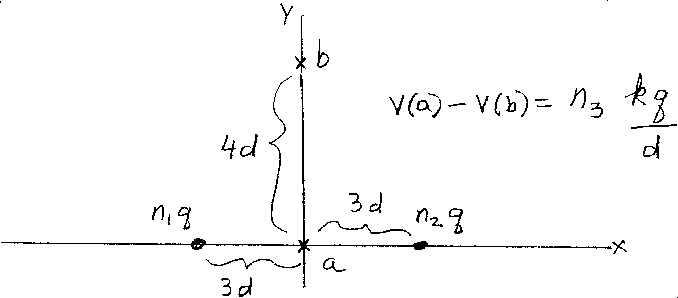Problem A7: In the figure above are two point objects. Both are located on the x-axis. One is located at (-3d,0) and has a charge of n1q. The other object is located at (+3d,0) and has a charge of n2q. Find the electrical potential difference between the points a and b located on the y-axis. Point a is at the origin (0,0), and point b is at (0,4d). What is n3 in the equation V(a)-V(b)=n3 kq/d ?n1 = n2 = Input n3:

If you are currently in my class, you can record your grade by entering your name and student ID number (without the leading zeros) below and clicking on "record grade".
 First Name = Last Name = ID = Problem: